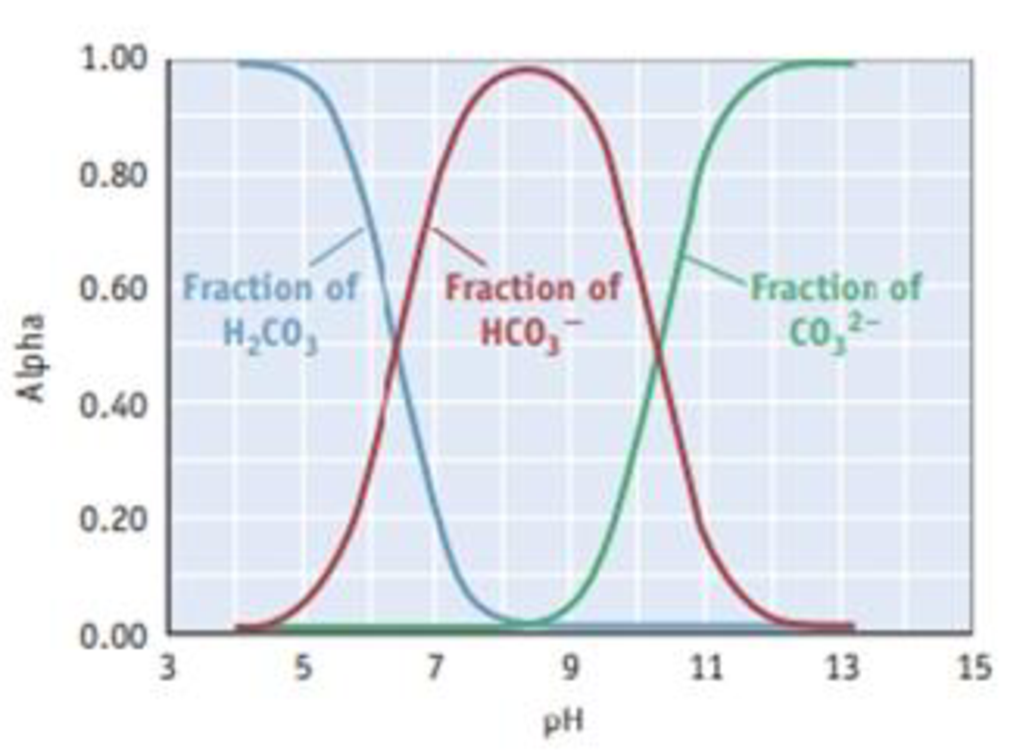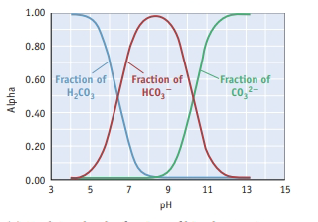# The composition diagram, or alpha plot, for the important acid-base system of carbonic acid, H 2 CO 3 , is illustrated. (See Study Question 1.7 for more information on such diagrams.) (a) Explain why the fraction of bicarbonate ion, HCO 3 − , rises and then falls as the pH increases. (b) What is the composition of the solution when the pH is 6.0? When the pH is 10.0? (c) If you wanted to buffer a solution at a pH of 11.0, what should be the ratio of HCO 3 − to CO 3 2− ?### Chemistry & Chemical Reactivity

9th Edition
John C. Kotz + 3 others
Publisher: Cengage Learning
ISBN: 9781133949640

#### Solutions

Chapter
Section### Chemistry & Chemical Reactivity

9th Edition
John C. Kotz + 3 others
Publisher: Cengage Learning
ISBN: 9781133949640
Chapter 17, Problem 118SCQ
Textbook Problem
5 views

## The composition diagram, or alpha plot, for the important acid-base system of carbonic acid, H2CO3, is illustrated. (See Study Question 1.7 for more information on such diagrams.)(a) Explain why the fraction of bicarbonate ion, HCO3−, rises and then falls as the pH increases. (b) What is the composition of the solution when the pH is 6.0? When the pH is 10.0? (c) If you wanted to buffer a solution at a pH of 11.0, what should be the ratio of HCO3− to CO32−?

a)

Interpretation Introduction

Interpretation:

The following by using the graph has to be determined.The fraction of bicarbonate ion, HCO3-, rises and then  falls as the pH increases has to be explained.

Concept introduction:

The pH is the unit of measurement for determining the acidity or alkalinity of a solution.

The mathematical definition of pH I negative logarithm of the molar hydrogen ion concentration is pH = -log[H+].

### Explanation of Solution

At very low pH levels, [H3O+] I very high. According to  Le Chateliers   the dissociation of H2CO3 is not favourable.  As pH increases, [H3O+] decreases , causing dissociation equilibrium of H2CO3 to favour the products . It makes the [HCO3-] increases initially.

As pH increases further, [H3O+] decrease even more

b)

Interpretation Introduction

Interpretation:

The following by using the graph has to be determined.1. (a) What is the composition of the solution when the pH is 6.0 and when the pH is 10.0.

c)

Interpretation Introduction

Interpretation:

The following by using the graph has to be determined.If you wanted to buffer a solution at a pH  of 11.0, the ratio of HCO3- to CO32- has to be predicted.

### Still sussing out bartleby?

Check out a sample textbook solution.

See a sample solution

#### The Solution to Your Study Problems

Bartleby provides explanations to thousands of textbook problems written by our experts, many with advanced degrees!

Get Started

Find more solutions based on key concepts
People most often choose foods for the nutrients they provide. T F

Nutrition: Concepts and Controversies - Standalone book (MindTap Course List)

Match each substance with its correct description

Human Biology (MindTap Course List)

A cyclotron (Fig. 28.16) designed to accelerate protons has an outer radius of 0.350 m. The protons are emitted...

Physics for Scientists and Engineers, Technology Update (No access codes included)

What element is found in DNA and RNA but not in proteins?

Introductory Chemistry: An Active Learning Approach

How is the ocean stratified by density? What names are given to the oceans density zones?

Oceanography: An Invitation To Marine Science, Loose-leaf Versin